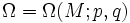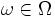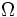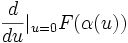# Derivative of functional on path space

Let$M$ be a differential manifold and$\Omega = \Omega(M;p,q)$ be a path space on$M$ (viz, the space of piecewise smooth paths from$p$ to$q$ in$M$.
Let$W$ be an element of the tangent space at a path$\omega \in \Omega$ and let$F$ be a functional from$\Omega$ to$\R$. Then the derivative of$F$ at the point$\omega \in \Omega$ with respect to the tangent direction$W$ is defined as follows:
Let$\alpha$ be any variation whose variation vector field is$W$. The derivative is then:$\frac{d}{du}|_{u=0} F(\alpha(u))$